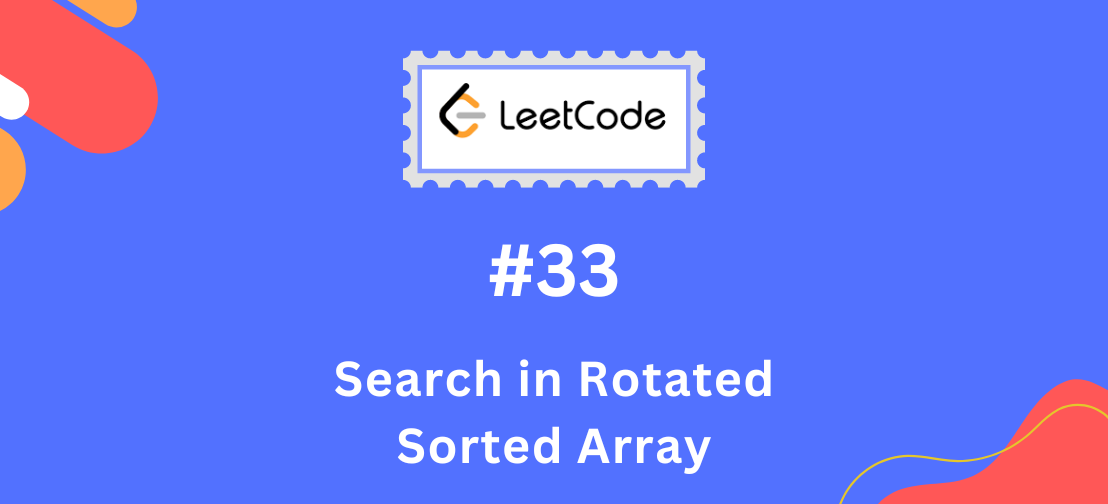# Leetcode 33 - Search in Rotated Sorted Array### Understanding the problem

LeetCode 33 is a problem where you are given a sorted array of integers that has been rotated by some number of elements, and a target value, and you need to find the target value in the array. Here is an example:

Input: nums = [4,5,6,7,0,1,2] target = 0

Output: 4

In this example, the array [4, 5, 6, 7, 0, 1, 2] was rotated 4 times to the right, so the element at index 0 is now at index 4.

### Grow Your Tech Career. Meet Expert coaches from top companies

Meet A MAANG Coach

To solve this problem, we can use a modified version of binary search. Since the array is sorted, we can use binary search to find the target value. However, since the array has been rotated, we need to consider the following two cases:

• If the middle element is greater than the element at the start of the array, then the start of the array is in the sorted half of the array.
• If the middle element is less than the element at the start of the array, then the end of the array is in the sorted half of the array.

We can use this information to determine which half of the array to search. If the target value is in the sorted half of the array, we can use binary search to find it. If the target value is not in the sorted half of the array, we can search the other half of the array.

Here is the implementation of the `search` function:

``````class Solution:
def search(self, nums: List[int], target: int) -> int:
# Set the start and end indices for the search
start = 0
end = len(nums) - 1
# Loop until the start and end indices meet or cross
while start <= end:
# Calculate the middle index
mid = (start + end) // 2
# If the target value is equal to the value at the middle index, return the middle index
if nums[mid] == target:
return mid
# If the middle element is greater than the element at the start of the array, the start of the array is in the sorted half
if nums[mid] > nums[start]:
# If the target value is in the sorted half, search the left half of the array
if target >= nums[start] and target < nums[mid]:
end = mid - 1
# If the target value is not in the sorted half, search the right half of the array
else:
start = mid + 1
# If the middle element is less than the element at the start of the array, the end of the array is in the sorted half
else:
# If the target value is in the sorted half, search the right half of the array
if target > nums[mid] and target <= nums[end]:
start = mid + 1
# If the target value is not in the sorted half, search the left half of the array
else:
end = mid - 1
return -1
``````

Five test cases that you can use to test the `search` function for solving LeetCode 33, the “Search in Rotated Sorted Array” problem.

``````# Test case 1
nums = [4, 5, 6, 7, 0, 1, 2]
target = 0
expected_output = 4
assert Solution().search(nums, target) == expected_output

# Test case 2
nums = [4, 5, 6, 7, 0, 1, 2]
target = 2
expected_output = 6
assert Solution().search(nums, target) == expected_output

# Test case 3
nums = [4, 5, 6, 7, 0, 1, 2]
target = 4
expected_output = 0
assert Solution().search(nums, target) == expected_output

# Test case 4
nums = [4, 5, 6, 7, 0, 1, 2]
target = 3
expected_output = -1
assert Solution().search(nums, target) == expected_output

# Test case 5
nums = [4, 5, 6, 7, 0, 1, 2]
target = 8
expected_output = -1
assert Solution().search(nums, target) == expected_output
``````

### Grow Your Tech Career. Meet Expert coaches from top companies

Meet A MAANG Coach

Related: